2016-11-03 10:31:40 u012042963 阅读数 2913
• ###### MATLAB图像处理

全面系统的学习MATLAB在图像处理中的应用

20781 人正在学习 去看看 魏伟2019-08-17 23:21:16 weixin_43211480 阅读数 159
• ###### MATLAB图像处理

全面系统的学习MATLAB在图像处理中的应用

20781 人正在学习 去看看 魏伟

### 一、一定要注意图像数据类型

im2double()函数

https://blog.csdn.net/weixin_43740956/article/details/89320146

2015-07-13 17:15:21 mikulee 阅读数 1112
• ###### MATLAB图像处理

全面系统的学习MATLAB在图像处理中的应用

20781 人正在学习 去看看 魏伟

## NDK 图像处理遇到的相关问题

1、SEGV_ACCERR

``````int testPixels(){
int len=640*480;
int pixels[len];
int index=0;
for(;index<len;index++){
pixels[index]=0xffff0000;
}
return 0;
}``````

``````int* pixels=NULL;
int testPixels(){
int len=640*480;
if(pixels==NULL){
//这里如果为空，就创建一个。暂时不考虑释放问题。
pixels=(int*)malloc(len*sizeof(int));
}
int index=0;
for(;index<len;index++){
pixels[index]=0xffff0000;
}
return 0;
}``````

2、JNI 中填充bitmap颜色不正确

``````int g_v_table,g_u_table,y_table;
int r_yv_table,b_yu_table;
int inited = 0;
void initTable()
{
if (inited == 0)
{
inited = 1;
int m = 0,n=0;
for (; m < 256; m++)
{
g_v_table[m] = 833 * (m - 128);
g_u_table[m] = 400 * (m - 128);
y_table[m] = 1192 * (m - 16);
}
int temp = 0;
for (m = 0; m < 256; m++)
for (n = 0; n < 256; n++)
{
temp = 1192 * (m - 16) + 1634 * (n - 128);
if (temp < 0) temp = 0; else if (temp > 262143) temp = 262143;
r_yv_table[m][n] = temp;

temp = 1192 * (m - 16) + 2066 * (n - 128);
if (temp < 0) temp = 0; else if (temp > 262143) temp = 262143;
b_yu_table[m][n] = temp;
}
}
}
jint Java_com_miku_jni_ImageUtilEngine_decodeYUV420SP(JNIEnv * env,
jobject thiz, jbyteArray buf, jobject bitmap, jint width, jint height) {
AndroidBitmapInfo info;
int* pixels = NULL;
if (AndroidBitmap_getInfo(env, bitmap, &info) >= 0) {
AndroidBitmap_lockPixels(env, bitmap, (void **) &pixels);
jbyte * yuv420sp = (*env)->GetByteArrayElements(env, buf, 0);
int frameSize = width * height;
initTable();
int i = 0, j = 0, yp = 0;
int uvp = 0, u = 0, v = 0;
for (j = 0, yp = 0; j < height; j++) {
uvp = frameSize + (j >> 1) * width;
u = 0;
v = 0;
for (i = 0; i < width; i++, yp++) {
int y = (0xff & ((int) yuv420sp[yp]));
if (y < 0)
y = 0;
if ((i & 1) == 0) {
v = (0xff & yuv420sp[uvp++]);
u = (0xff & yuv420sp[uvp++]);
}

int y1192 = y_table[y];
int r = r_yv_table[y][v];
int g = (y1192 - g_v_table[v] - g_u_table[u]);
int b = b_yu_table[y][u];

if (g < 0)
g = 0;
else if (g > 262143)
g = 262143;
//原文这里如果直接赋值给bitmap的像素的话就有问题(并不是函数有问题)，
//因为bitmap的数  据是RGBARGBA这样的序列
//但这里pixels[yp]为整形，数据格式是(小端格式)LittleEndian
//直接赋值一个整数，例如pixels[yp]=0xff051003(ff为A,05为R,10为G,03为B)的话，
//在内存中是这样的存储的[031005ff],并不是你直接赋的值[ff051003]。对比[031005ff]
//和RGBA顺序，03(B)和05(R)的位置刚好倒置了，所以在机器上，显示的颜色就不正确了。
//pixels[yp] = 0xff000000 | ((r << 6) & 0xff0000)
//      | ((g >> 2) & 0xff00) | ((b >> 10) & 0xff);
//改为下面，颜色即可正确
pixels[yp] = 0xff000000 | ((b << 6) & 0xff0000)
| ((g >> 2) & 0xff00) | ((r >> 10) & 0xff);
}
}
(*env)->ReleaseByteArrayElements(env, buf, yuv420sp, 0);
AndroidBitmap_unlockPixels(env, bitmap);
}
return 1;
}``````

3、FFmpeg相关
FFmpeg编译可以参考这位大神的编译指南FFmpeg的Android平台移植，我编译的是当前最新的2.7.1版本，按步骤来，能正常编译出各个so库文件。

``````static {
//先按顺序加ffmpeg动态库
//最后才加载自己写的库
}``````

2019-06-16 22:04:48 weixin_44210987 阅读数 28
• ###### MATLAB图像处理

全面系统的学习MATLAB在图像处理中的应用

20781 人正在学习 去看看 魏伟

0.错误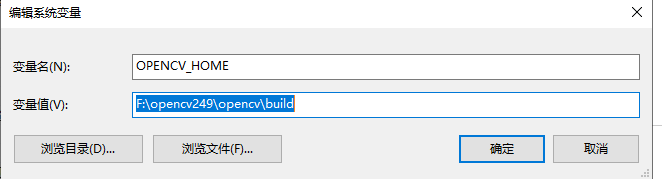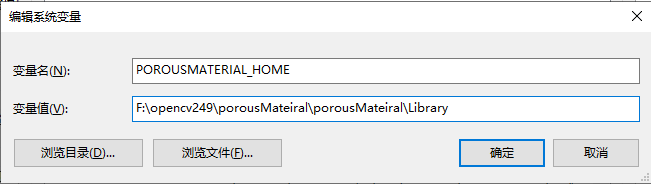1. 错误

1 error LNK2001: 无法解析的外部符号 "public: virtual struct CRuntimeClass * __thiscall PictureDialog::GetRuntimeClass(void)const " (?GetRuntimeClass@PictureDialog@@UBEPAUCRuntimeClass@@XZ) F:\BianCheng\MacthineVision\PorousCharacteristicAnalysis\PorousCharacteristicAnalysis\PictureDialog.obj

<1>,在.h文件中写了DECLARE_DYNAMIC，而在.cpp文件中没有写IMPLEMENT_DYNAMIC
<2>,在.h文件中写了DECLARE_DYNCREATE ,但在.cpp文件中没有写上IMPLEMENT_DYNCREATE

1. 错误
错误 1 error LNK2001: 无法解析的外部符号 “protected: virtual void __thiscall PictureDialog::DoDataExchange(class CDataExchange *)” (?DoDataExchange@PictureDialog@@MAEXPAVCDataExchange@@@Z) F:\BianCheng\MacthineVision\PorousCharacteristicAnalysis\PorousCharacteristicAnalysis\PictureDialog.obj

3.错误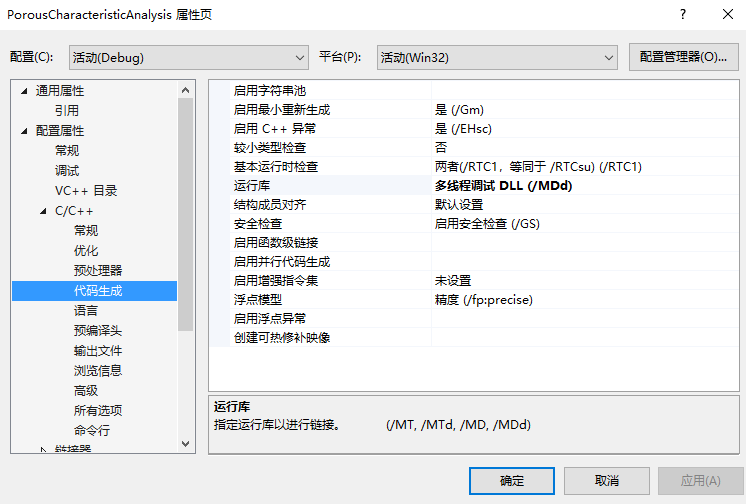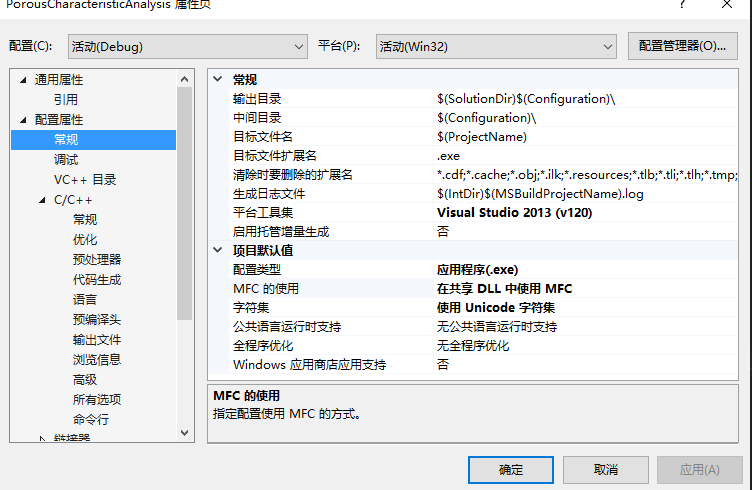4.错误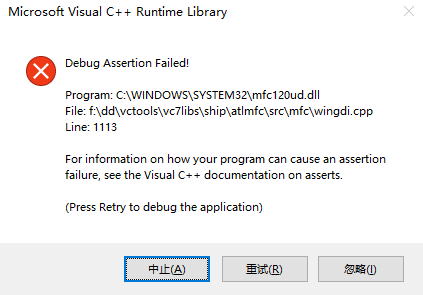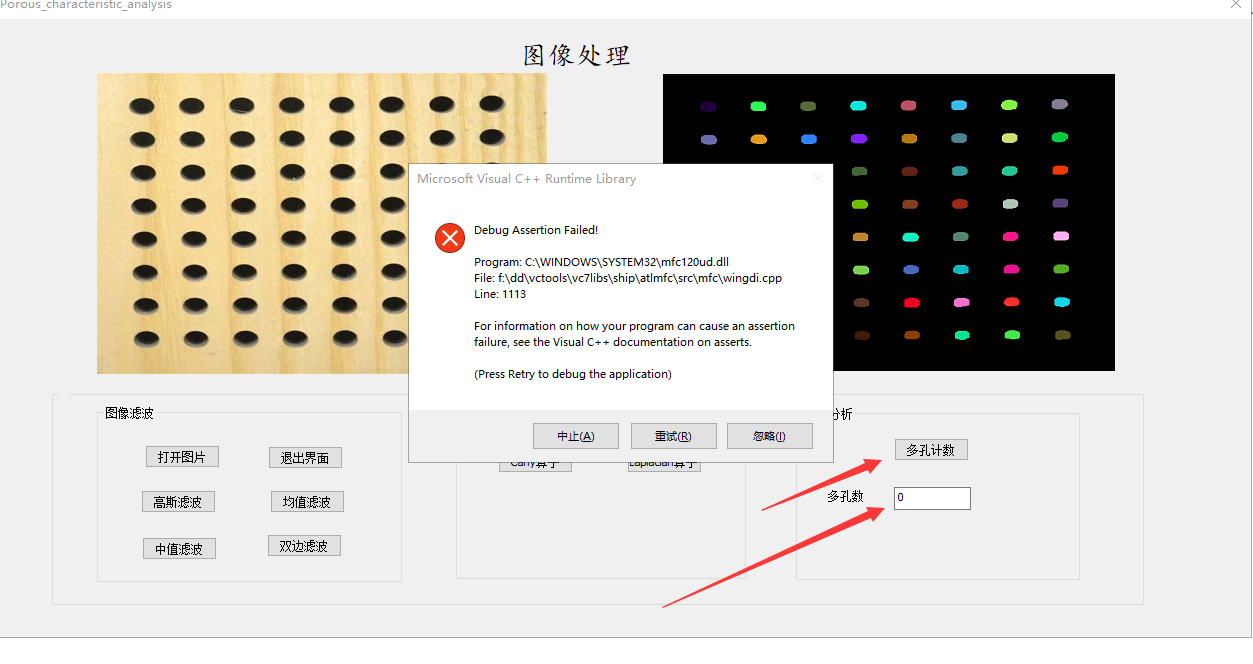``````    // 将控件中的数据保存到相应的变量
UpdateData(TRUE);

// 将被点数赋值给m_editSummand
m_editSummand = contours.size();

// 根据各变量的值更新相应的控件。和的编辑框会显示m_editSum的值
UpdateData(FALSE);
``````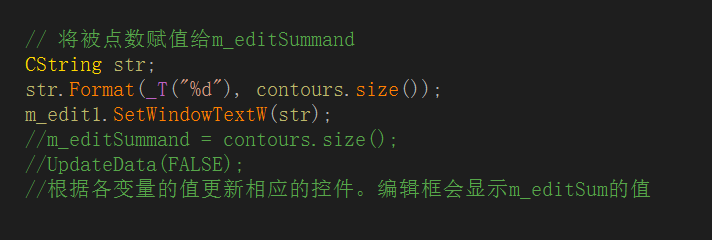2017-12-08 23:15:24 weixin_36297465 阅读数 283
• ###### MATLAB图像处理

全面系统的学习MATLAB在图像处理中的应用

20781 人正在学习 去看看 魏伟

# python图像处理过程中遇到的坑

## 使用PIL进行图像的处理:

• 使用PIL.open()方法打开一张图片,此时图片是一个图像对象,不是numpy数组.
• 此时这个图像的对象可以进行各种操作, 例如灰度,提高亮度,增强, 调整分辨率等等.
• 使用np.array功能,可以将一个图像对象转化为np的数组. 然后用于神经网络或者其他的各种操作.
• 在使用PIL的fromarray功能时候,很可能会遇到bug,提示”格式不支持”.这个时候很有可能是mode没有选择正确.
• 在使用fromarray 功能时候, 我们要保证数组中的值都是在0到255之间, np.asarray(np.clip(derained, 0, 255), dtype=”uint8”).
• 此时主要用到的就是函数clip可以将一个数组中的数值全部局限在后面两个值之间.

## 除了PIL, 我们还可以使用matplotlib

• 使用matplotlib的一个好处是可以使用pylab来进行图片的保存,同时可以直接从numpy数组绘制整个图片.